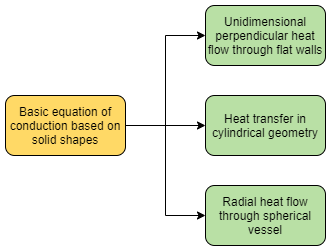## What is Convection and Its Problem Example

In previous post we learned what is conduction and its example on heat loss through a pipe wall with insulation. In this post, I want to share what is convection and its problem example.

Convection is heat transfer between a solid and an adjacent fluids develops as a result of fluid molecular movement. Cold molecules replace hot molecules as they leave the solid surface. A thin layer or film next to the solid surface is where most of the resistance to this type of heat transfer occurs. Even though the bulk fluid flow is extremely turbulent, this layer still exists.

Convective heat transport is governed by Newton’s law of cooling.

Q = h ∙A∙ ∆T

Where:

Q = heat transfer (Btu/hr)

h = heat transfer coefficient [Btu/(hr∙ ft2oF)]

A = area (ft2)

∆T = temperature difference (oF)

Convective heat transfer is divided into two types, natural or free convection and forced convection. Read More## What is Conduction and Its Problem Example

In this post I want to share what is conduction and its problem example.

In contrast to general molecular motion or mixing, conduction describes the rate of heat transfer through materials as a function of vibrations and interactions between nearby molecules. Conduction always applies to solids and rarely to fluids.

There are several fundamental equations for steady heat conduction through some basic solid shapes, neglecting conditions of border:Read More